GFG App
Open AppBrowser
Continue

Christiaan Huygens proposed the Huygens principle. In 1678, he changed the way we think about light and its properties. You’ve probably heard of the rectilinear theory of light, which states that light travels in straight lines. One of the most important ways for examining various optical phenomena is the Huygens principle. The principle is an analysis method that can be used to solve wave propagation problems in the far-field limit, near-field diffraction, and reflection.

When you open a window in a room, light comes in through it and spreads around the space. Do you know why this happens? This is due to the fact that light has a wave character and spreads around the room in all directions. Let’s look at Huygen’s Principle to get a better understanding of this.

### Huygen’s Principle

Every point on a wavefront is a source of spherical wavelets that expand out at the speed of light in the forward direction. The wavefront is formed by the sum of these spherical wavelets.”

This explanation, however, does not explain why refraction took happened in the first place. Second, it was unable to explain how light transfers energy along its path. The Huygens Principle, also known as the Huygens–Fresnel Principle, emphasises the wave propagation behaviour as follows:

• Secondary sources generate wavelets that are comparable to the original source’s wavelets.
• The new wavefront is determined by the common tangent on the wavelets in the forward direction at any given time.
• The total of the spherical wavelets is the wavefront.

### Wavefront and Wave Normal

Consider a point source of light ‘S’ in ‘air.’ The light waves emitted by this source go in all directions. If ‘c’ is the velocity of light in air, each wave will travel ‘ct’ in time t and reach the surface of a sphere with radius ‘ct’. With the source ‘S’ as its focal point. This type of surface is known as a spherical wave surface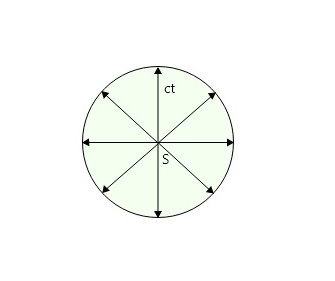Wave surface

A wavefront is a locus of all the points of medium to which waves arrive simultaneously so that all the points are in the same phase (see image below), for example, light emitted by a bulb at a limited distance. A plane wavefront emerges from a convex lens when a point source of light is positioned at its focal point. In addition, if the spherical wavefront is sufficiently big, a tiny portion of the surface can be considered a plane wavefront. For example, wavefronts are caused by sunlight. A linear source (such as a slit) produces a cylindrical wavefront. For example, light is emitted by a fluorescent tube.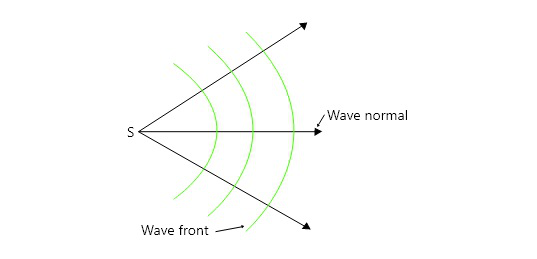Wavefront and Wavenormal

A wave normal is a perpendicular drawn to the surface of a wavefront in the direction of light propagation. A wavefront, in other words, transports light energy perpendicular to the surface.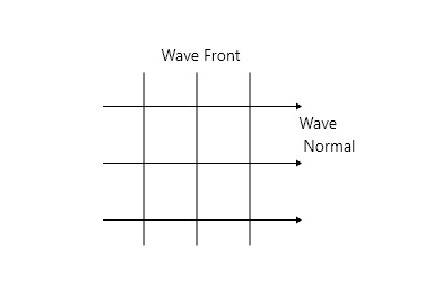Wave Normal

Types of wavefronts – There are three types of wavefronts, that are

1. Spherical wavefront: Consider the ‘S’ point source of light. Light waves generated by S can move in any direction. If c is the speed of light, then each wave will reach the surface of a sphere with radius ct and centre S after time t. This spherical surface is known as the spherical wavefront at time t, and it is derived from a finite-distance point source. For example, consider the light emitted by a bulb at a limited distance.
2. Plane wavefront: The spherical wavefront is so enormous at a very vast distance from the point source that a tiny portion of it may be considered a plane wavefront. It is derived from an infinitely distant point source. As an example, consider the wavefront caused by sunlight.
3. Cylindrical wavefront: If the source of light is linear i.e., a slit, then we get a cylindrical wavefront. It is obtained from a linear source kept at a finite distance. For example, light is emitted by a fluorescent tube.

### Huygen’s Construction of a spherical wavefront

Let PQ be a cross-section of a spherical wavefront due to a point source(S), at any instant. This can be called as primary wavefront. Now consider points A, B, C, D, E on PQ. They act as secondary sources and send out secondary wavelets as per Huygens’ principle.

If c is the speed of light in the isotropic medium, in time t, each wave will describe a distance ‘ct’. With A, B, C, D, E as centres of circles, each radius ct will be traced. Each circle will represent a secondary wavefront.

The common tangential surface (envelope) P’Q’ drawn to these secondary wavefronts represents the (new) position of the wavefront after time ‘t’. The secondary waves moving in the backward direction do not exist.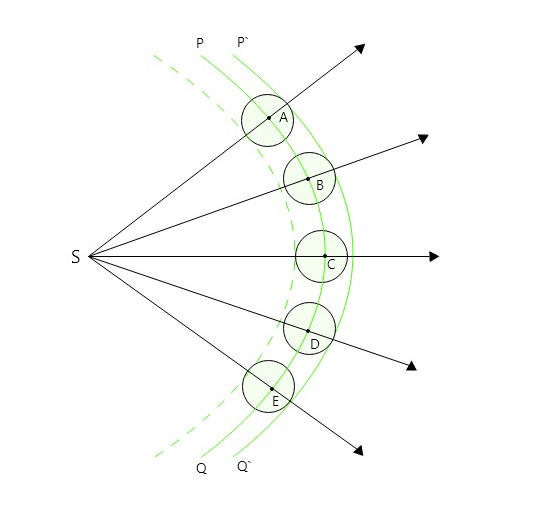Huygen’s Spherical Wavefront

### Huygen’s Construction of a plane wavefront

Let PQ be a plane wavefront perpendicular to the plane of the paper due to a point source(S), at any instant and at a very large distance, This can be called a primary wavefront. Now consider points A, B, C, D on PQ. They act as secondary Huygens’ sources and send out secondary wavelets as per Huygens’ principle.

If ‘c’ is the speed of light in the isotropic medium, in time ‘t’, each wave will describe a distance ‘ct’. With A, B, C, D as centres, spheres each of radius ‘ct will be traced, each sphere will represent a secondary wavefront. The common tangential surface(envelope) i.e.P’Q’ drawn to these secondary wavefronts represents the new position for the wavefront after time ‘t’.

The secondary waves moving in the backward direction do not exist and therefore they are shown with dotted lines.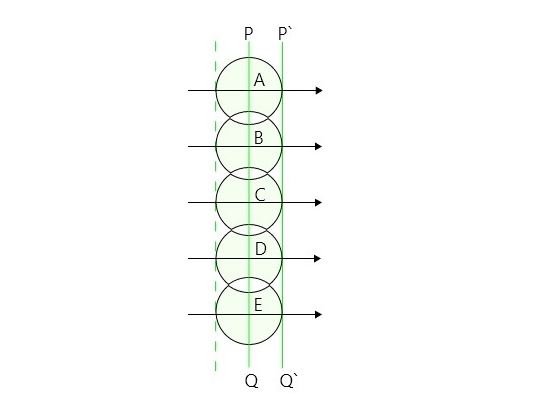Huygen’s plane wavefront

• It could explain the laws of refraction, interference, reflection, diffraction, etc.
• The wave theory predicted that the speed of light in an optically denser medium is less than that in an optically rarer medium as was experimentally proved by Focault.

• It could not explain the rectilinear propagation of light. The Rectilinear Propagation of Light describes that Light travels in a Straight Line.
• It could not explain the phenomenon of polarization of light and phenomena like the Raman effect, Compton effect and Photoelectric effect. It was modified later that light is a transverse wave.
• Huygens`assumed the presence of a hypothetical medium [Ether]. The properties of hypothetical ether were studied further by Michelson and Morley with their apparatus based on the principles of interference of light beams. The experiment concluded that there is no ether drag when the earth moved through it. This and other experiments proved that ether does not exist.

### Sample Questions

Question 1: State any three assumptions of Huygens wave theory of light?

Assumptions of Huygens wave theory are as below:

1. Light is propagated in form of longitudinal waves
2. Different colors of light are due to different wavelengths of light waves.
3. We feel sensation when light waves enter our eyes.

Question 2: State merits and Demerits of Huygens wave theory of light

Merits:

• This theory could explain the laws of reflection, refraction, diffraction, interference, etc.
• According to this theory, the speed of light in a denser medium is less than that in a rarer medium. [This conclusion is in perfect agreement with the experimental results.]

Demerits:

• His theory could not explain rectilinear propagation of light.
• Huygens` assumed the presence of the hypothetical medium, ether.
• Experimentally it was proved that ether does not exist.
• This theory fails to explain the polarization of light phenomenon like Photoelectric effect, Compton effect and Raman effect.

Question 3: Define Wavefront and Wave Normal.

Wavefront: A locus containing all of the points of medium to which waves arrive at the same time, so that all of the points are in the same phase.

Wave normal: A wave normal is a perpendicular drawn to the surface of a wavefront at any point along the wavefront in the direction of light propagation.

Question 4: Give the difference between Plane and Spherical wavefront?

The difference between Plane and Spherical wavefront are:

Question 5: Explain how cylindrical wavefronts are produced and give an example?